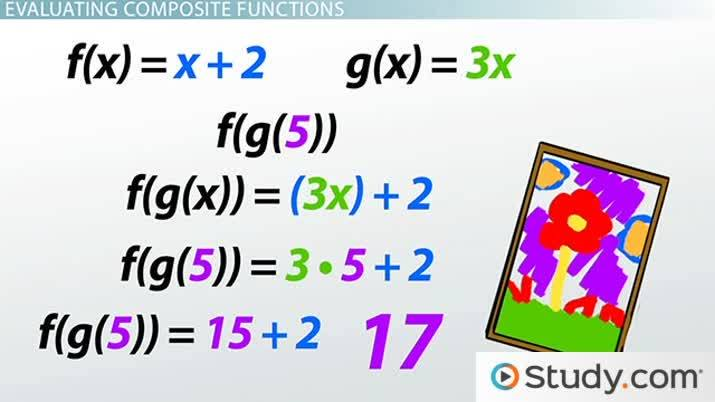# How to Evaluate Composite Functions

Lesson Transcript
Instructor: Tyler Cantway

Tyler has tutored math at two universities and has a master's degree in engineering.

Multiple mathematic functions can be combined into a composite function where the value of one function depends on the other. Learn how to write, define, and evaluate composite functions with a series of examples. Updated: 08/26/2021

## Painting in Art Class

When each person was painting one color, they got the same answer. But when Ashley painted first and Brad painted second, the final poster might get a completely different color depending on the colors they used.An error occurred trying to load this video.

Try refreshing the page, or contact customer support.

Coming up next: Manipulating Functions and Solving Equations for Different Variables

### You're on a roll. Keep up the good work!

Replay
Your next lesson will play in 10 seconds
• 0:05 Painting in Art Class
• 0:58 Defining Composite Functions
• 1:22 Writing Composite Functions
• 2:54 Evaluating Composite Functions
• 4:11 Lesson Summary
Save Save

Want to watch this again later?

Timeline
Autoplay
Autoplay
Speed Speed

## Defining Composite Functions

In math we have special formulas, called functions, that tell us an answer when we plug in a specific number. However, like the paintings, we can put functions together so that one function gives us a different answer depending on the answer of another. Composite functions use the output of one function as the input of another. This is like a function within a function.

## Writing Composite Functions

Let's look at two normal functions: f(x) = x + 2 and g(x) = 3x. If we wanted to make a composite function, we would have to put one function inside the other. To make a composite function where we put g(x) inside the function f(x), we can write it f(g(x)).

Notice that instead of simply putting an x in the function, we substitute the entire g(x) function. When we do that, we have f(g(x)) = (3x) + 2. On the left, you'll see that the g function is inside the f function. On the right, you'll see that instead of the x we substituted 3x.

We can do this other ways too. We could substitute the f function inside the g function. When you put the f function inside the g function, you get g(f(x)) = 3(x+2).

You can even make a composite function of itself. We can make f(f(x)) = (x+2) +2 or g(g(x)) = 3(3x). In each case, we take the whole function and substitute it where we see x.

To unlock this lesson you must be a Study.com Member.

### Register to view this lesson

Are you a student or a teacher?